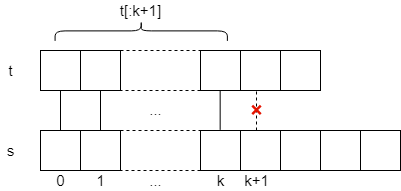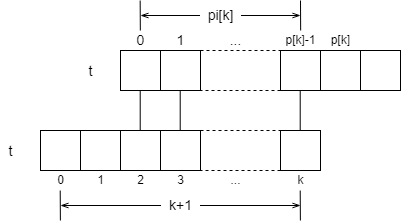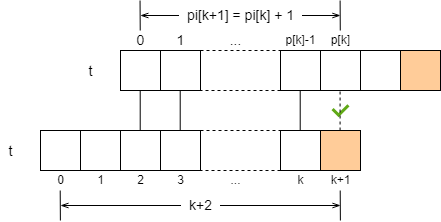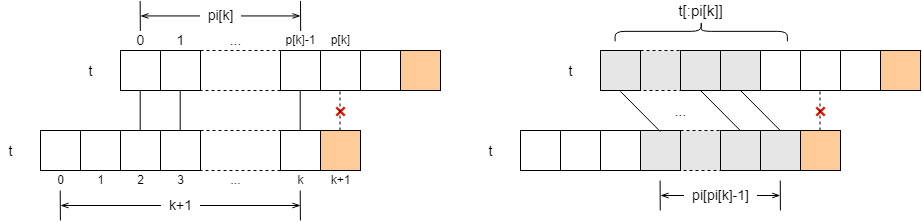String matching is a very common algorithm. What it does is, for the pattern string `t`, find the first occurrence of it in the target string `s`. For example, if `t = "abcab", s = "ababcabd"`, the algorithm should return 2. Talking about string matching, we have to mention the KMP algorithm. KMP is a very powerful algorithm, which is very clever and can match strings in linear time complexity. In this article, we’ll learn about it.

This article will use Python’s slicing syntax to represent substrings. If you’re not familiar with Python, just remember that `s[:k]` means that `s` is prefixed by `k` in length, and `s[-k:]` means that `s` is suffixed by `k` in length. In addition, the subscript of the string starts from 0, so the last character of `s[:k]` is `s[k-1]`.

### Matching algorithm

Roughly speaking, the KMP algorithm exploits a property of strings: it is possible for the end of a string to match its own first part. For example, the string `abcab` has two characters at the end that can match its own first part:

 ``````1 2 3 `````` `````` abcab || abcab ``````

In formal language: For a string `t`, there exists a maximum number `0 <= m < len(t)` such that `t[:m] == t[-m:]` holds. We call `m` the maximum first and last match length. Note that `m` must be less than the length of `t`, otherwise it is meaningless – any string is always equal to itself.

KMP first finds the maximum first and last match lengths for all prefixes of the pattern string, which are stored in the array `pi`. The maximum first and last match length for the prefix `t[:i]` is `pi[i-1]`. For example, the `pi` array for the string `p = "abcab"` is `[0, 0, 0, 1, 2]`, because:

• `p[:1] = "a"`, the maximum first and last match length is 0, `pi = 0`
• `p[:2] = "ab"`, maximum first and last match length is 0, `pi = 0`
• `p[:3] = "abc"`, maximum first and last match length is 0, `pi = 0`
• `p[:4] = "abca"`, the maximum first and last match length is 1, `pi = 1`, because there is
 ``````1 2 3 `````` `````` abca | abca ``````
• `p[:5] = "abcab"`, the maximum first and last match length is 2, `pi = 2`, because
 ``````1 2 3 `````` `````` abcab || abcab ``````

Let’s not worry about how KMP finds the `pi` array, but let’s see how KMP uses the `pi` array to do matching.

Suppose the string `s` matches the pattern string `t`. We start with the 0th character, then the 1st, 2nd, and up to the kth character, and the match is successful; however, the kth + 1th character fails.The match failed, so what do we do? Here’s the kicker. The first 0 to k characters match successfully, and the substring equals `t[:k+1]` . The `pi` array tells us that the last `pi[k]` characters of this substring are exactly equal to the first `pi[k]` characters of it.So next we let `s[k+1]` compare with `t[pi[k]]`, if it’s equal, we continue to match the next characters; if it’s not equal - no matter, `t[:pi[k]]` has already matched, we go back to the `pi` array to get the maximum length of the first and last matches of this substring, and compare the corresponding characters in the same way.

With this in mind, it is easy to write the code for the KMP algorithm:

 `````` 1 2 3 4 5 6 7 8 9 10 11 12 `````` ``````def kmp(s, t): pi = calc_pi(t) # 先不管如何计算 pi j = 0 for i, c in enumerate(s): while j > 0 and t[j] != c: # t[j] 匹配失败, 但是 t[:j] 匹配成功 j = pi[j-1] # t[:j] 的后 pi[j-1] 个字符与前 pi[j-1] 个字符相等, 下一步匹配 t[pi[j-1]] if t[j] == c: j += 1 if j == len(t): return i - j + 1 # 返回起始位置 return -1 ``````

### Find the pi array

Since the `pi` array is so useful, how do we find it? First it’s easy to think of `pi = 0` , because the maximum first and last match length needs to be less than the string length. If we can use `pi, pi, ... , pi[k]` pushes out `pi[k+1]` , we can find the entire `pi` array.

Suppose we find `pi[k]` , which is the maximum first and last match length of `t[:k+1]`.What is the maximum first match length of `pi[k+1]`, i.e., `t[:k+2]`? This is discussed in two cases. Suppose `t[k+1] == t[pi[k]]` , which is a simple case, `pi[k+1] = pi[k] + 1` .What if they’re not equal? That’s fine, the first few characters, `t[:pi[k]]`, match, don’t they? Based on the `pi` array we just found, we know that for the substring `t[:pi[k]]`, the last `pi[pi[k]-1]` characters are equal to the first `pi[pi[k]-1]` characters! This brings us back to the previous case of the matching algorithm.Next we just need to compare `t[k+1]` with `t[pi[pi[k]-1]]`. If they are equal, then `pi[k+1] = pi[pi[k]-1] + 1`; if they are not equal, then we repeat the operation: query the `pi` array to get the maximum first and last matches of the previous equal parts, and then compare the corresponding characters.

In this way, the code for computing the `pi` array is easy to understand.

 `````` 1 2 3 4 5 6 7 8 9 10 11 `````` ``````def calc_pi(t): pi =  * len(t) # pi = 0 j = 0 # j 等于 pi[-1] for i in range(1, len(t)): while j > 0 and t[i] != t[j]: # t[j] 匹配失败, 但是 t[:j] 匹配成功. 注意这里 j = pi[i-1] j = pi[j-1] # t[:j] 的后 pi[j-1] 个字符与前 pi[j-1] 个字符相等, 下一步匹配 t[pi[j-1]] if t[i] == t[j]: j += 1 pi[i] = j return pi ``````

The code to compute `pi` is very similar to the KMP matching algorithm. The matching algorithm matches the pattern string with the target string, while computing `pi` matches the pattern string with itself. The matching process only relies on the previously computed `pi` value, so it is a dynamic programming algorithm.

### Summary

The following code puts the two parts of the algorithm together. To recap, KMP uses the matching algorithm to gradually derive the `pi` array, and then uses the same matching algorithm to match the pattern string with the target string using the previously derived `pi`. So the KMP algorithm is a very powerful algorithm.

 `````` 1 2 3 4 5 6 7 8 9 10 11 12 13 14 15 16 17 18 19 20 21 `````` ``````def kmp(s, t): if not t: return 0 pi =  * len(t) j = 0 for i in range(1, len(t)): while j > 0 and t[i] != t[j]: j = pi[j-1] if t[i] == t[j]: j += 1 pi[i] = j j = 0 for i, c in enumerate(s): while j > 0 and t[j] != c: j = pi[j-1] if t[j] == c: j += 1 if j == len(t): return i - j + 1 return -1 ``````